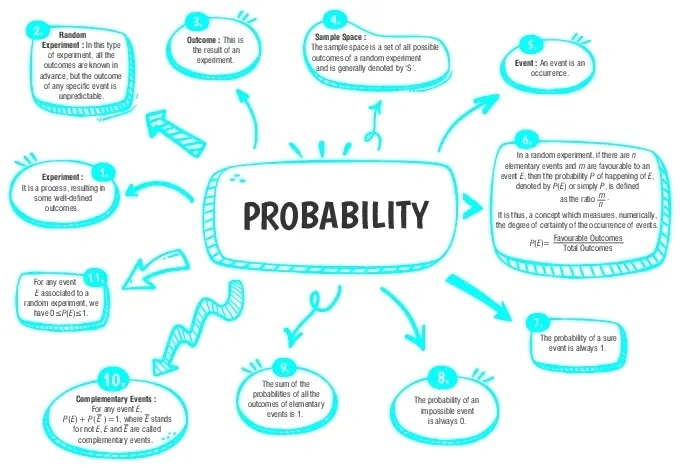Probability

# Class 10 Math Chapter 14 Probability Important QuestionsHere are some essential questions for Class 10 Mathematics Chapter 15, Probability, thoughtfully chosen to aid students in their preparation for the CBSE Class 10 Mathematics Examination in 2023-24. By practicing these varied problems, students can enhance their comprehension of Probability concepts and refine their problem-solving skills.

## Introduction

In Chapter 14 of Class 10 Mathematics, Probability, we will delve into the Classical Definition of Probability. This concept allows us to quantify the likelihood of an event occurring by comparing the number of favorable outcomes to the total possible outcomes. Additionally, we will solve simple problems to determine the probability of specific events.

### What is probability?

Probability is a measure that quantifies the likelihood of an event occurring. It is expressed as a value between 0 and 1, where 0 represents an impossible event, and 1 represents a certain event. Probability helps us understand and predict outcomes in various situations.### Class 10 Statistics Important Questions and Answers

#### (a)$$\frac{1}{13}$$(b)$$\frac{1}{4}$$(c)$$\frac{12}{13}$$(d)$$\frac{3}{4}$$

Ans. (c)$$\frac{12}{13}$$

Explanation:
Total number of possible outcomes = 52
Number of aces in the pack = 4
Thus, the probability of not drawing an ace
∴ P(E) =$$\frac{52-4}{52}$$ =$$\frac{12}{13}$$

#### (a)$$\frac{2}{5}$$(b)$$\frac{3}{5}$$(c)$$\frac{1}{10}$$(d)None of these

Ans. (b)$$\frac{3}{5}$$

Explanation:
Total number of possible outcomes = 10
The total number of favourable outcomes = 6
∴ P(E) = $$\frac{6}{10}$$ = $$\frac{3}{5}$$

#### Q3. A card is drawn from a well shuffled pack of 52 playing cards. Find the probability that the drawn card is neither a king nor a queen.

Ans. probability that the drawn card is neither a king nor a queen
$$=\frac{11}{13}$$

Explanation:
The number of cards =52
Number of kings and queens=4+4=8
Thus, number of favorable outcomes
= 52−8 = 44
∴  P(E) = $$\frac{44}{52}=\frac{11}{13}$$

#### Q4. A card is drawn at random from a shuffled pack of 52 cards. Find the probability of drawing a (i) face card, and (ii) a card which is neither a king nor a red card.

Ans. The probability of drawing a (i) face card
$$=\frac{3}{13}$$
and (ii) a card which is neither a king nor a red card
$$=\frac{6}{13}$$

Explanation:
∴  P(E) =$$\frac{12}{52}=\frac{3}{13}$$
(ii) The number of favourable outcomes of drawing neither a king nor a red card
= 52 – (2 + 26)
= 24
∴   P(E)=$$\frac{24}{52}=\frac{6}{13}$$

#### Q5. A bag contains 6 black balls and some white balls. If the probability of drawing a white ball from the bag is double that of a black ball, find the number of white balls in the bag.

Ans. The number of white balls = 12.

Explanation:
Let the number of white balls be x.
Thus, the total number of the possible outcomes
= 6 + x
Now, the favourable outcomes if the black ball is drawn = 6
∴   P(B) =$$\frac{6}{6+x}$$
Again, the favourable outcomes if the white ball is drawn = x
∴   P(W) = $$\frac{6}{6+x}$$
According to the question,
$$\frac{6}{6+x}=2\begin{pmatrix} \frac{6}{6+x} \end{pmatrix}$$
⇒ x = 12
Hence, the number of white balls = 12.#### CBSE Class 10 Maths Chapter wise Important Questions

Chapter No. Chapter Name
Chapter 1 Real Number
Chapter 2 Polynomials
Chapter 3 Pair of Linear Equations in Two Variables
Chapter 5 Arithmetic Progressions
Chapter 6 Triangles
Chapter 7 Coordinate Geometry
Chapter 8 Introduction to Trigonometry
Chapter 9 Some Applications of Trigonometry
Chapter 10 Circles
Chapter 11 Areas Related to Circle
Chapter 12 Surface Areas and Volumes
Chapter 13 Statistics
Chapter 14 Probability

#### Conclusion

To improve your understanding of the Probability chapter, you can explore oswal.io. This platform offers a range of practice questions designed to make learning easier. By practicing these questions, you can strengthen your grasp of probability concepts and enhance your skills in solving probability-related problems.

#### Q1 : How is the probability of an event calculated?

Ans: The probability of an event is calculated by dividing the number of favorable outcomes by the total possible outcomes.

#### Q2 : What is the sum of the probabilities of all possible outcomes in an experiment?

Ans: The sum of the probabilities of all possible outcomes in an experiment is equal to 1.

#### Q3 : How can you find the probability of an event not occurring?

Ans: The probability of an event not occurring is calculated by subtracting the probability of the event occurring from 1.

#### Q4 : What does a probability of 0.5 represent?

Ans: A probability of 0.5 represents an event that is equally likely to occur or not to occur.

#### Q5 : Define an "event" in probability terms.

Ans: An event is a specific outcome or set of outcomes in an experiment or situation.

###### Copyright 2022 OSWAL PUBLISHERS Simplifying ExamsPhone:  (+91) 78959 87722
Mail: support@oswalpublishers.in